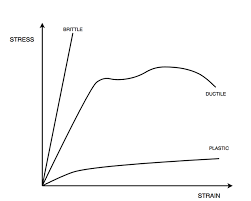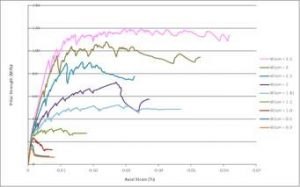## How to Calculate and Solve for Young’s Modulus | Rock MechanicsThe image above represents young’s modulus.

To compute for young’s modulus, two essential parameters are needed and these parameters are axial stress (σa) and axial strain (εa).

The formula for calculating the young’s modulus:

E = σa / εa

Where:

E = Young’s Modulus
σa = Axial Stress
εa = Axial Strain

Let’s solve an example;
Given that the axial stress is 65 and the axial strain is 45. Calculate for the young’s modulus?

This implies that;

σa = Axial Stress = 65
εa = Axial Strain = 45

E = σa / εa
E = 65 / 45
E = 1.44

Therefore, the young’s modulus is 1.44.

Calculating the Axial Stress when the Young’s Modulus and the Axial Strain is Given.

σa = E x εa

Where;

σa = Axial Stress
E = Young’s Modulus
εa = Axial Strain

Let’s solve an example;
Given that the young’s modulus is 25 and the axial strain is 10. Calculate for the axial stress?

This implies that;

E = Young’s Modulus = 25
εa = Axial Strain = 10

σa = E x εa
σa = 25 x 10
σa = 250

Therefore, the axial stress is 250.

## How to Calculate and Solve for Axial Strain | Rock MechanicsThe image above represents axial strain.

To compute for axial strain, two essential parameters are needed and these parameters are change in length (ΔL) and length (L).

The formula for calculating the axial strain:

εo = ΔL / L

Where;

εo = Axial Strain
ΔL = Change in Length
L = Total Length

Let’s solve an example;
Calculate the axial strain when the change in length is 18 and total length is 32.

This implies that;

ΔL = Change in Length = 18
L = Total Length = 32

εo = ΔL / L
εo = 18 / 32
εo = 0.5625

Therefore, the axial strain is 0.5625.

Calculating the Change in Length when the Axial Strain and Total Length is Given.

ΔL = εo x L

Where;

ΔL = Change in Length
εo = Axial Strain
L = Total Length

Let’s solve an example;
Calculate the change in length when the axial strain is 21 and total length is 9

This implies that;

εo = Axial Strain = 21
L = Total Length = 9

ΔL = εo x L
ΔL = 21 x 9
ΔL = 189

Therefore, the change in length is 189.